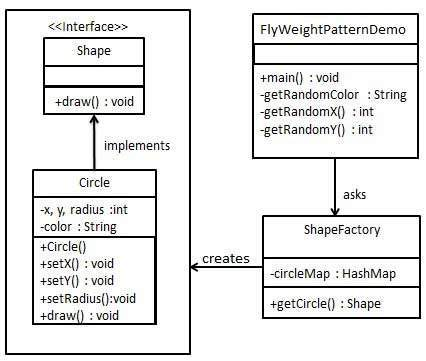# 享元模式

### 介绍

**优点：**大大减少对象的创建，降低系统的内存，使效率提高。

### 实现

ShapeFactory 有一个 CircleHashMap，其中键名为 Circle 对象的颜色。无论何时接收到请求，都会创建一个特定颜色的圆。ShapeFactory 检查它的 HashMap 中的 circle 对象，如果找到 Circle 对象，则返回该对象，否则将创建一个存储在 hashmap 中以备后续使用的新对象，并把该对象返回到客户端。

FlyWeightPatternDemo，我们的演示类使用 ShapeFactory 来获取 Shape 对象。它将向 ShapeFactory 传递信息（red / green / blue/ black / white），以便获取它所需对象的颜色。#### 步骤 1

Shape.java

public interface Shape {

void draw();

}


#### 步骤 2

Circle.java

public class Circle implements Shape {

private String color;
private int x;
private int y;

public Circle(String color) {
this.color = color;
}

public void setColor(String color) {
this.color = color;
}

public void setX(int x) {
this.x = x;
}

public void setY(int y) {
this.y = y;
}

}

@Override
public void draw() {
System.out.println("Circle: Draw() [Color : " + color
+ ", x : " + x + ", y : " + y + ", radius : " + radius);
}

}


#### 步骤 3

ShapeFactory.java

public class ShapeFactory {

private static final Map<String, Shape> circleMap = new HashMap<>();

public static Shape getCircle(String color) {
Circle circle = (Circle) circleMap.get(color);
if (circle == null) {
circle = new Circle(color);
circleMap.put(color, circle);
System.out.println("Creating circle of color : " + color);
}
return circle;
}

}


#### 步骤 4

FlyweightPatternDemo.java

public class FlyweightPatternDemo {

private static final String[] colors =
{"Red", "Green", "Blue", "White", "Black"};

public static void main(String[] args) {

for (int i = 0; i < 20; ++i) {
Circle circle = (Circle) ShapeFactory.getCircle(getRandomColor());
circle.setX(getRandomX());
circle.setY(getRandomY());
circle.draw();
System.out.println();
}
}

private static String getRandomColor() {
return colors[(int) (Math.random() * colors.length)];
}

private static int getRandomX() {
return (int) (Math.random() * 100);
}

private static int getRandomY() {
return (int) (Math.random() * 100);
}

}


#### 步骤 5

Creating circle of color : White
Circle: Draw() [Color : White, x : 56, y : 48, radius : 100

Circle: Draw() [Color : White, x : 80, y : 65, radius : 100

Circle: Draw() [Color : White, x : 54, y : 75, radius : 100

Creating circle of color : Red
Circle: Draw() [Color : Red, x : 3, y : 10, radius : 100

Creating circle of color : Blue
Circle: Draw() [Color : Blue, x : 78, y : 0, radius : 100

Circle: Draw() [Color : Red, x : 85, y : 77, radius : 100

Creating circle of color : Green
Circle: Draw() [Color : Green, x : 1, y : 69, radius : 100

Circle: Draw() [Color : Red, x : 45, y : 27, radius : 100

Circle: Draw() [Color : Green, x : 52, y : 48, radius : 100

Creating circle of color : Black
Circle: Draw() [Color : Black, x : 81, y : 71, radius : 100

Circle: Draw() [Color : Red, x : 75, y : 89, radius : 100

Circle: Draw() [Color : Black, x : 78, y : 12, radius : 100

Circle: Draw() [Color : Green, x : 11, y : 43, radius : 100

Circle: Draw() [Color : Black, x : 28, y : 20, radius : 100

Circle: Draw() [Color : Green, x : 64, y : 84, radius : 100

Circle: Draw() [Color : Red, x : 65, y : 72, radius : 100

Circle: Draw() [Color : Red, x : 30, y : 65, radius : 100

Circle: Draw() [Color : Black, x : 50, y : 36, radius : 100

Circle: Draw() [Color : Green, x : 87, y : 77, radius : 100

Circle: Draw() [Color : Red, x : 41, y : 18, radius : 100

01-281503
09-2016606-0437
01-281077
03-206757
04-214964
07-151022